Publicité

# Lecture007.pdf

21 Aug 2022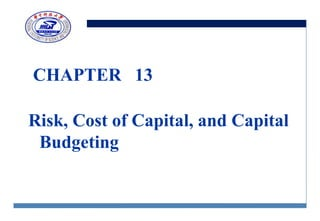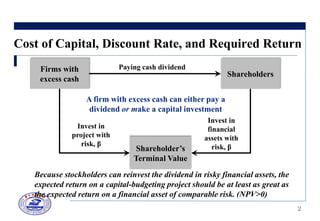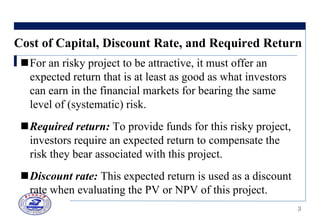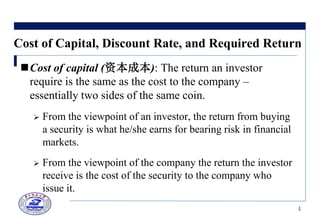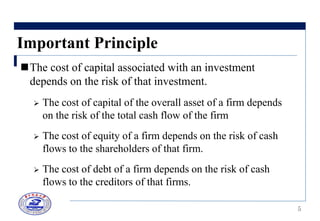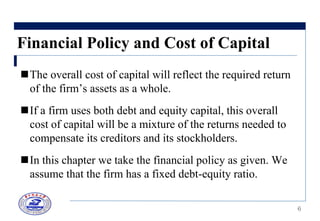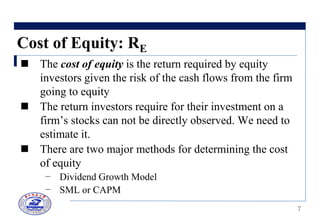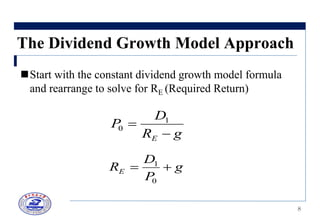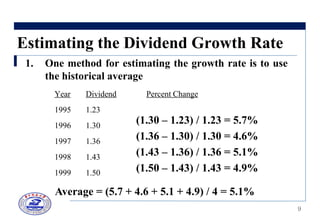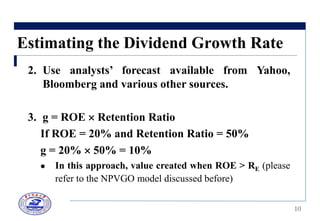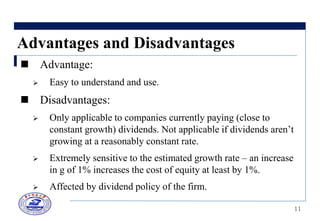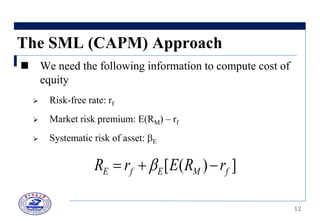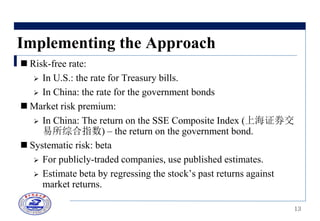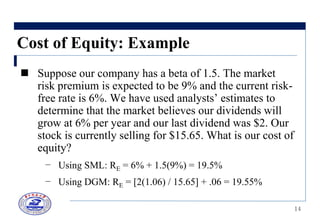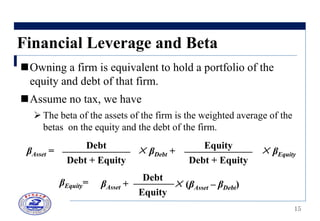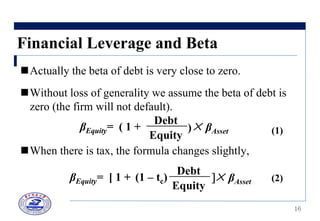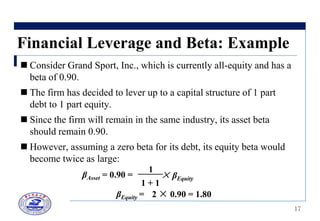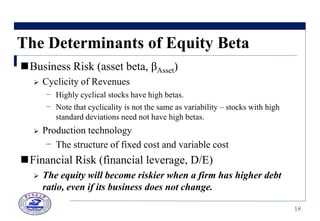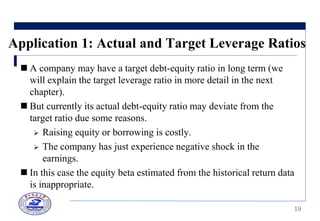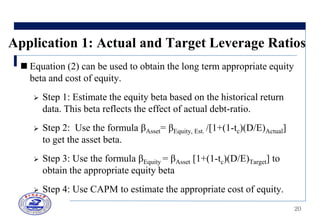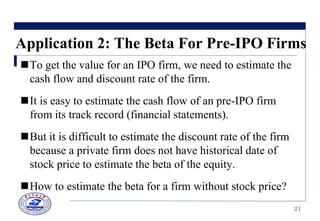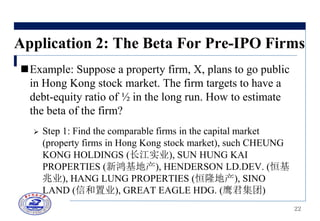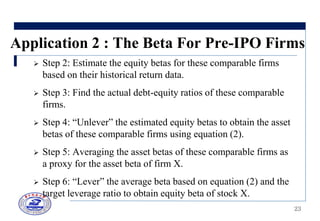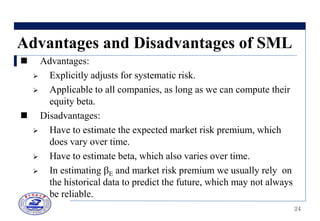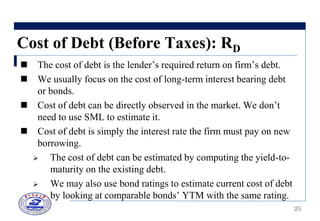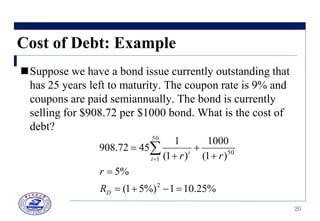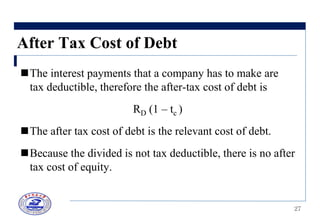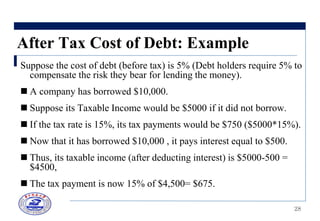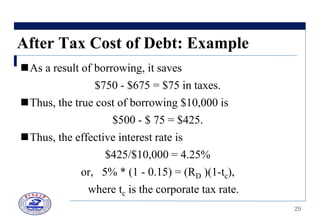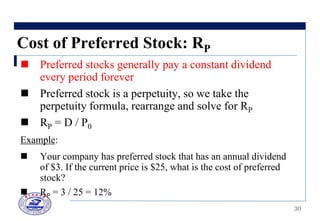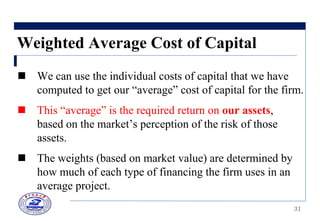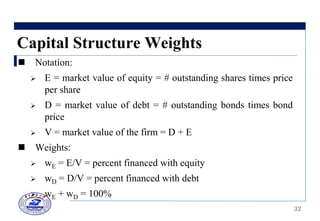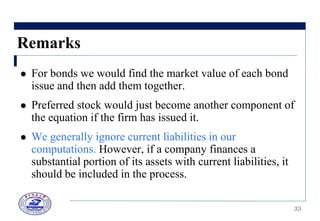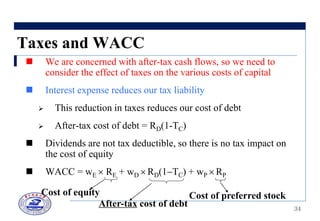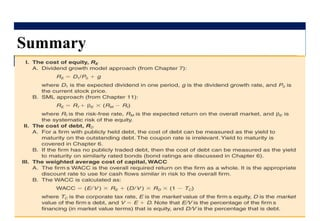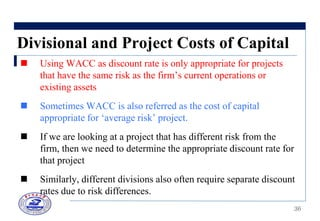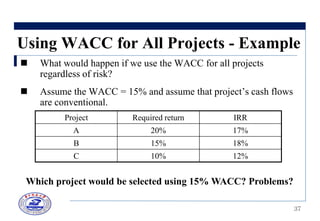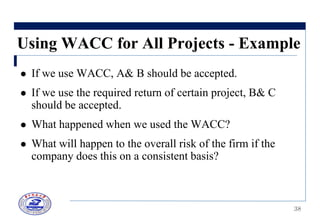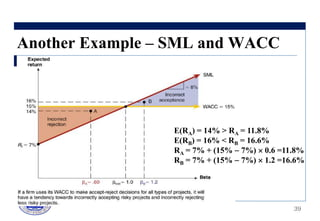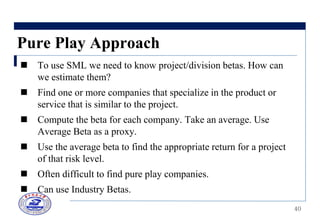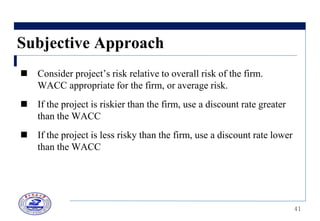1 sur 41
Publicité

### Lecture007.pdf

1. CHAPTER 13 Risk, Cost of Capital, and Capital Budgeting
2. 2 Cost of Capital, Discount Rate, and Required Return Firms with excess cash Shareholders Paying cash dividend Shareholder’s Terminal Value Invest in project with risk, β Invest in financial assets with risk, β A firm with excess cash can either pay a dividend or make a capital investment Because stockholders can reinvest the dividend in risky financial assets, the expected return on a capital-budgeting project should be at least as great as the expected return on a financial asset of comparable risk. (NPV>0)
3. 3 Cost of Capital, Discount Rate, and Required Return For an risky project to be attractive, it must offer an expected return that is at least as good as what investors can earn in the financial markets for bearing the same level of (systematic) risk. Required return: To provide funds for this risky project, investors require an expected return to compensate the risk they bear associated with this project. Discount rate: This expected return is used as a discount rate when evaluating the PV or NPV of this project.
4. 4 Cost of Capital, Discount Rate, and Required Return Cost of capital (资本成本): The return an investor require is the same as the cost to the company – essentially two sides of the same coin.  From the viewpoint of an investor, the return from buying a security is what he/she earns for bearing risk in financial markets.  From the viewpoint of the company the return the investor receive is the cost of the security to the company who issue it.
5. 5 Important Principle The cost of capital associated with an investment depends on the risk of that investment.  The cost of capital of the overall asset of a firm depends on the risk of the total cash flow of the firm  The cost of equity of a firm depends on the risk of cash flows to the shareholders of that firm.  The cost of debt of a firm depends on the risk of cash flows to the creditors of that firms.
6. 6 Financial Policy and Cost of Capital The overall cost of capital will reflect the required return of the firm‟s assets as a whole. If a firm uses both debt and equity capital, this overall cost of capital will be a mixture of the returns needed to compensate its creditors and its stockholders. In this chapter we take the financial policy as given. We assume that the firm has a fixed debt-equity ratio.
7. 7 Cost of Equity: RE  The cost of equity is the return required by equity investors given the risk of the cash flows from the firm going to equity  The return investors require for their investment on a firm‟s stocks can not be directly observed. We need to estimate it.  There are two major methods for determining the cost of equity – Dividend Growth Model – SML or CAPM
8. 8 The Dividend Growth Model Approach Start with the constant dividend growth model formula and rearrange to solve for RE (Required Return) g R D P E   1 0 g P D RE   0 1
9. 9 Estimating the Dividend Growth Rate 1. One method for estimating the growth rate is to use the historical average Year Dividend Percent Change 1995 1.23 1996 1.30 1997 1.36 1998 1.43 1999 1.50 (1.30 – 1.23) / 1.23 = 5.7% (1.36 – 1.30) / 1.30 = 4.6% (1.43 – 1.36) / 1.36 = 5.1% (1.50 – 1.43) / 1.43 = 4.9% Average = (5.7 + 4.6 + 5.1 + 4.9) / 4 = 5.1%
10. 10 Estimating the Dividend Growth Rate 2. Use analysts’ forecast available from Yahoo, Bloomberg and various other sources. 3. g = ROE  Retention Ratio If ROE = 20% and Retention Ratio = 50% g = 20%  50% = 10%  In this approach, value created when ROE > RE (please refer to the NPVGO model discussed before)
11. 11 Advantages and Disadvantages  Advantage:  Easy to understand and use.  Disadvantages:  Only applicable to companies currently paying (close to constant growth) dividends. Not applicable if dividends aren‟t growing at a reasonably constant rate.  Extremely sensitive to the estimated growth rate – an increase in g of 1% increases the cost of equity at least by 1%.  Affected by dividend policy of the firm.
12. 12 The SML (CAPM) Approach  We need the following information to compute cost of equity  Risk-free rate: rf  Market risk premium: E(RM) – rf  Systematic risk of asset: E ] ) ( [ f M E f E r R E r R    
13. 13 Implementing the Approach  Risk-free rate:  In U.S.: the rate for Treasury bills.  In China: the rate for the government bonds  Market risk premium:  In China: The return on the SSE Composite Index (上海证券交 易所综合指数) – the return on the government bond.  Systematic risk: beta  For publicly-traded companies, use published estimates.  Estimate beta by regressing the stock‟s past returns against market returns.
14. 14 Cost of Equity: Example  Suppose our company has a beta of 1.5. The market risk premium is expected to be 9% and the current risk- free rate is 6%. We have used analysts‟ estimates to determine that the market believes our dividends will grow at 6% per year and our last dividend was \$2. Our stock is currently selling for \$15.65. What is our cost of equity? – Using SML: RE = 6% + 1.5(9%) = 19.5% – Using DGM: RE = [2(1.06) / 15.65] + .06 = 19.55%
15. 15 Financial Leverage and Beta Owning a firm is equivalent to hold a portfolio of the equity and debt of that firm. Assume no tax, we have  The beta of the assets of the firm is the weighted average of the betas on the equity and the debt of the firm. βAsset = Debt + Equity Debt × βDebt + Debt + Equity Equity × βEquity βEquity= βAsset + Equity Debt × (βAsset – βDebt)
16. 16 Financial Leverage and Beta Actually the beta of debt is very close to zero. Without loss of generality we assume the beta of debt is zero (the firm will not default). When there is tax, the formula changes slightly, βEquity= ( 1 + Equity Debt × βAsset ) βEquity= [ 1 + × βAsset Equity Debt ] (1 – tc) (1) (2)
17. 17 Financial Leverage and Beta: Example  Consider Grand Sport, Inc., which is currently all-equity and has a beta of 0.90.  The firm has decided to lever up to a capital structure of 1 part debt to 1 part equity.  Since the firm will remain in the same industry, its asset beta should remain 0.90.  However, assuming a zero beta for its debt, its equity beta would become twice as large: βAsset = 0.90 = 1 + 1 1 × βEquity βEquity = 2 × 0.90 = 1.80
18. 18 The Determinants of Equity Beta Business Risk (asset beta, βAsset)  Cyclicity of Revenues – Highly cyclical stocks have high betas. – Note that cyclicality is not the same as variability – stocks with high standard deviations need not have high betas.  Production technology – The structure of fixed cost and variable cost Financial Risk (financial leverage, D/E)  The equity will become riskier when a firm has higher debt ratio, even if its business does not change.
19. 19 Application 1: Actual and Target Leverage Ratios  A company may have a target debt-equity ratio in long term (we will explain the target leverage ratio in more detail in the next chapter).  But currently its actual debt-equity ratio may deviate from the target ratio due some reasons.  Raising equity or borrowing is costly.  The company has just experience negative shock in the earnings.  In this case the equity beta estimated from the historical return data is inappropriate.
20. 20 Application 1: Actual and Target Leverage Ratios  Equation (2) can be used to obtain the long term appropriate equity beta and cost of equity.  Step 1: Estimate the equity beta based on the historical return data. This beta reflects the effect of actual debt-ratio.  Step 2: Use the formula βAsset= βEquity, Est. /[1+(1-tc)(D/E)Actual] to get the asset beta.  Step 3: Use the formula βEquity = βAsset [1+(1-tc)(D/E)Target] to obtain the appropriate equity beta  Step 4: Use CAPM to estimate the appropriate cost of equity.
21. 21 Application 2: The Beta For Pre-IPO Firms To get the value for an IPO firm, we need to estimate the cash flow and discount rate of the firm. It is easy to estimate the cash flow of an pre-IPO firm from its track record (financial statements). But it is difficult to estimate the discount rate of the firm because a private firm does not have historical date of stock price to estimate the beta of the equity. How to estimate the beta for a firm without stock price?
22. 22 Application 2: The Beta For Pre-IPO Firms Example: Suppose a property firm, X, plans to go public in Hong Kong stock market. The firm targets to have a debt-equity ratio of ½ in the long run. How to estimate the beta of the firm?  Step 1: Find the comparable firms in the capital market (property firms in Hong Kong stock market), such CHEUNG KONG HOLDINGS (长江实业), SUN HUNG KAI PROPERTIES (新鸿基地产), HENDERSON LD.DEV. (恒基 兆业), HANG LUNG PROPERTIES (恒隆地产), SINO LAND (信和置业), GREAT EAGLE HDG. (鹰君集团)
23. 23 Application 2 : The Beta For Pre-IPO Firms  Step 2: Estimate the equity betas for these comparable firms based on their historical return data.  Step 3: Find the actual debt-equity ratios of these comparable firms.  Step 4: “Unlever” the estimated equity betas to obtain the asset betas of these comparable firms using equation (2).  Step 5: Averaging the asset betas of these comparable firms as a proxy for the asset beta of firm X.  Step 6: “Lever” the average beta based on equation (2) and the target leverage ratio to obtain equity beta of stock X.
24. 24 Advantages and Disadvantages of SML  Advantages:  Explicitly adjusts for systematic risk.  Applicable to all companies, as long as we can compute their equity beta.  Disadvantages:  Have to estimate the expected market risk premium, which does vary over time.  Have to estimate beta, which also varies over time.  In estimating E and market risk premium we usually rely on the historical data to predict the future, which may not always be reliable.
25. 25 Cost of Debt (Before Taxes): RD  The cost of debt is the lender‟s required return on firm‟s debt.  We usually focus on the cost of long-term interest bearing debt or bonds.  Cost of debt can be directly observed in the market. We don‟t need to use SML to estimate it.  Cost of debt is simply the interest rate the firm must pay on new borrowing.  The cost of debt can be estimated by computing the yield-to- maturity on the existing debt.  We may also use bond ratings to estimate current cost of debt by looking at comparable bonds‟ YTM with the same rating.
26. 26 Cost of Debt: Example Suppose we have a bond issue currently outstanding that has 25 years left to maturity. The coupon rate is 9% and coupons are paid semiannually. The bond is currently selling for \$908.72 per \$1000 bond. What is the cost of debt? % 25 . 10 1 %) 5 1 ( % 5 ) 1 ( 1000 ) 1 ( 1 45 72 . 908 2 50 1 50            D i i R r r r
27. 27 After Tax Cost of Debt The interest payments that a company has to make are tax deductible, therefore the after-tax cost of debt is RD (1 – tc ) The after tax cost of debt is the relevant cost of debt. Because the divided is not tax deductible, there is no after tax cost of equity.
28. 28 After Tax Cost of Debt: Example Suppose the cost of debt (before tax) is 5% (Debt holders require 5% to compensate the risk they bear for lending the money).  A company has borrowed \$10,000.  Suppose its Taxable Income would be \$5000 if it did not borrow.  If the tax rate is 15%, its tax payments would be \$750 (\$5000*15%).  Now that it has borrowed \$10,000 , it pays interest equal to \$500.  Thus, its taxable income (after deducting interest) is \$5000-500 = \$4500,  The tax payment is now 15% of \$4,500= \$675.
29. 29 After Tax Cost of Debt: Example As a result of borrowing, it saves \$750 - \$675 = \$75 in taxes. Thus, the true cost of borrowing \$10,000 is \$500 - \$ 75 = \$425. Thus, the effective interest rate is \$425/\$10,000 = 4.25% or, 5% * (1 - 0.15) = (RD )(1-tc), where tc is the corporate tax rate.
30. 30 Cost of Preferred Stock: RP  Preferred stocks generally pay a constant dividend every period forever  Preferred stock is a perpetuity, so we take the perpetuity formula, rearrange and solve for RP  RP = D / P0 Example:  Your company has preferred stock that has an annual dividend of \$3. If the current price is \$25, what is the cost of preferred stock?  RP = 3 / 25 = 12%
31. 31 Weighted Average Cost of Capital  We can use the individual costs of capital that we have computed to get our “average” cost of capital for the firm.  This “average” is the required return on our assets, based on the market‟s perception of the risk of those assets.  The weights (based on market value) are determined by how much of each type of financing the firm uses in an average project.
32. 32 Capital Structure Weights  Notation:  E = market value of equity = # outstanding shares times price per share  D = market value of debt = # outstanding bonds times bond price  V = market value of the firm = D + E  Weights:  wE = E/V = percent financed with equity  wD = D/V = percent financed with debt  wE + wD = 100%
33. 33 Remarks  For bonds we would find the market value of each bond issue and then add them together.  Preferred stock would just become another component of the equation if the firm has issued it.  We generally ignore current liabilities in our computations. However, if a company finances a substantial portion of its assets with current liabilities, it should be included in the process.
34. 34 Taxes and WACC  We are concerned with after-tax cash flows, so we need to consider the effect of taxes on the various costs of capital  Interest expense reduces our tax liability  This reduction in taxes reduces our cost of debt  After-tax cost of debt = RD(1-TC)  Dividends are not tax deductible, so there is no tax impact on the cost of equity  WACC = wE  RE + wD  RD(1TC) + wP  RP Cost of preferred stock Cost of equity After-tax cost of debt
35. 35 Summary
36. 36 Divisional and Project Costs of Capital  Using WACC as discount rate is only appropriate for projects that have the same risk as the firm‟s current operations or existing assets  Sometimes WACC is also referred as the cost of capital appropriate for „average risk‟ project.  If we are looking at a project that has different risk from the firm, then we need to determine the appropriate discount rate for that project  Similarly, different divisions also often require separate discount rates due to risk differences.
37. 37 Using WACC for All Projects - Example  What would happen if we use the WACC for all projects regardless of risk?  Assume the WACC = 15% and assume that project‟s cash flows are conventional. Project Required return IRR A 20% 17% B 15% 18% C 10% 12% Which project would be selected using 15% WACC? Problems?
38. 38 Using WACC for All Projects - Example  If we use WACC, A& B should be accepted.  If we use the required return of certain project, B& C should be accepted.  What happened when we used the WACC?  What will happen to the overall risk of the firm if the company does this on a consistent basis?
39. 39 E(RA) = 14% > RA = 11.8% E(RB) = 16% < RB = 16.6% RA = 7% + (15%  7%)  0.6 =11.8% RB = 7% + (15%  7%)  1.2 =16.6% Another Example – SML and WACC
40. 40 Pure Play Approach  To use SML we need to know project/division betas. How can we estimate them?  Find one or more companies that specialize in the product or service that is similar to the project.  Compute the beta for each company. Take an average. Use Average Beta as a proxy.  Use the average beta to find the appropriate return for a project of that risk level.  Often difficult to find pure play companies.  Can use Industry Betas.
41. 41 Subjective Approach  Consider project‟s risk relative to overall risk of the firm. WACC appropriate for the firm, or average risk.  If the project is riskier than the firm, use a discount rate greater than the WACC  If the project is less risky than the firm, use a discount rate lower than the WACC
Publicité